# MAP 7th Grade Math : Statistics and Probability

## Example Questions

### Example Question #1 : Statistics And Probability

Kelly spun a spinner, which is pictured below,times. She recorded her results in the table provided. What is the experimental probability of the spinner landing on the yellow section?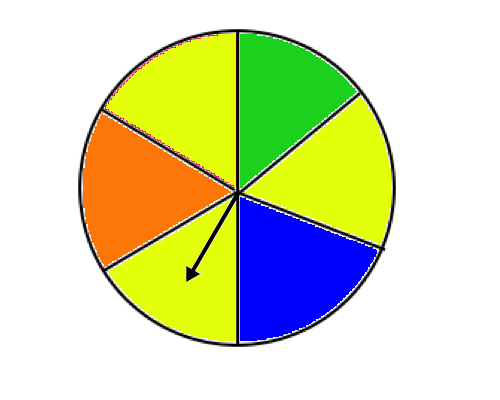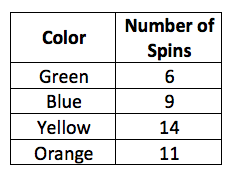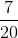Explanation:

The experimental probability is what actually happened in an experiment. In this case, Kelly spun the spinnertimes, and she landed on yellow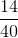times; thus, our experimental probability is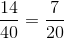### Example Question #2 : Statistics And Probability

Samantha has a bag of marbles containingpink marbles,orange marble,red marbles,purple marbles,white marbles,black marble,green marbles, andyellow marble. What is the probability that Samantha will pick a red marble out of the bag?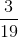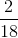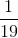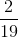Explanation:

In this problem we have a total ofmarbles. That means that each marble, regardless of color, has achance of being picked, as shown in the images below. This image shows equal probability because each marble has achance of being drawn, which is equal to the probability of all of the other marbles.Samantha wants to know what her probability is of drawing a red marble out of her bag. Remember, probability is the number of favorable outcomes over the total number of outcomes: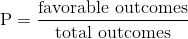For this problem there arered marbles; therefore,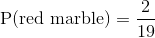### Example Question #1 : Statistics And Probability

A university wanted to compare the heights of women on the basketball team and women on the swim team. The data is in the dot plots provided.Using the dot plots provided, what is the mean absolute deviation of the height of basketball players, rounded to the nearest tenth?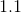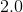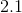Explanation:

The mean absolute deviation is the average distance between each data point and the mean. So the first step to solving for the mean absolute deviation is to solve for the mean.

The mean is the average of a data set. In order to solve for the mean, we add all of our heights together, and divide by the number of people in our data set.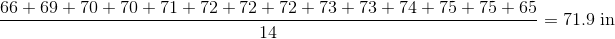Next, we subtract to find the distance between each data point and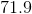, which is shown in the following table: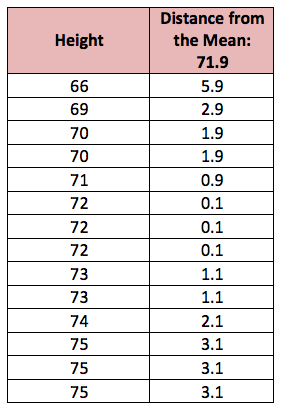Now that we have all of the distances from the mean, we find the average of those differences to find the mean absolute deviation: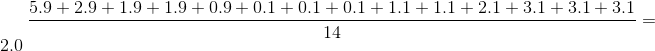### All MAP 7th Grade Math Resources